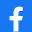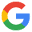CREAR TESTUSUARIO PASSWORD Recordarme [Olvidé mis datos]

REALIZAR TEST
 Título del test: Inglés unidad 4 Descripción: 2ª Eva. unidad 4 Autor:Libre(Otros tests del mismo autor)Fecha de Creación: 07/03/2023 Categoría: Idiomas Número preguntas: 20
 Comparte el test:Comparte el test:Últimos Comentarios
No hay ningún comentario sobre este test.
Temario:
 The device used for measuring energy is called Multimeter Wattmeter Ohmmeter Energy meter.The power factor of an installation can be determined by using: A voltmeter and an ammeter A wattmeter A power meter A phase meter.A wattmeter has been placed between phases 1 and 2 of a 3-phase balanced system. The value of electric powerin this installation will be 3 times the power measure displayed by the wattmeter 2 times the power given by the wattmeter Two more measures are needed to calculate power. A varmeter would be needed for measuring power in a 3-phase system.A wattmeter measures, in AC systems, Apparent power Reactive power Active power None.The resistance of a multimeter when measuring electric currents will be: As high as possible, so as not to distort the measurement As low as possible, so as not to distort the measurement A multimeter has no internal resistance.The measurement of electric current is done with: A wattmeter A voltmeter An ohmmeter An ammeter.Voltage (electric potential) is measured : By series connecting the multimeter and the load By series connecting the ammeter to the load By connecting the multimeter in parallel to the load Electric potential cannot be measured.The resistance featured by a multimeter when measuring voltage will be: As high as possible, so as not to distort the measurement As low as possible, so as not to distort the measurement A multimeter has no internal resistance.A clamp meter can be used for: Measuring weak alternate current magnitudes Measuring weak direct current magnitudes Measuring high alternate current magnitudes None.When a conductor’s continuity needs to be known…: An ammeter can be used to check that current goes through the conductor A voltmeter can be used to check that there is potential difference between both ends An ohmmeter can be used to measure the resistance between both ends of the conductor A multimeter can be used in the “Continuity” mode.The mean value of a sinusoidal wave with maximum value of 15V is: 7,5 V 10,6V 9,5 V 8V.A multimeter can be used for measuring: Just voltage Just current Several electric variables All.The efficient value of a sinusoidal wave with maximum value of 10V is: 10V 14,14V 7,07V 6,36V.According to the REBT, specifically to the ITC-BT 03, some of the technical means utilised by the authorisedinstallers of the basic category are: Networks, harmonics and network disturbances analyser Tester of operating theatre watchers and AC 3-phase power-energy analyser-recorder Earth resistance meter, isolation meter and multimeter Lux meter, ground isolation measuring electrodes and multimeter.An oscilloscope has shown that the period of a sinusoidal wave amounts to 10 ms. The frequency of this wave willbe: 0,1 Hz 10Hz 100Hz 1000Hz.The tester of differential breaker trigger sensitivity… Is not required for an authorised installer Is only required for authorised installers of the specialist category Is only required for authorised installers of the basic category Is required for any authorised installer.In order to measure electric currents, the device must be connected: In series with the load In parallel with the load Electric currents can only be measured with clamp meters.The unit of measurement of power is: KW/h W/s Watt Joule.The representation of a magnitude will depend upon: That this magnitude is analogical That this magnitude is digital The type of device utilised None.The measurement of power can be done with a: Wattmeter Voltmeter and ohmmeter Voltmeter and ammeter Both a and c are correct.
 Denunciar test Condiciones de uso
INICIO
CREAR TEST
COMENTARIOS